Vibroengineering PROCEDIA

Published: 19 October 2019

# Dynamics similarity design and verification of rotor system

Lijia Chen1
Guihuo Luo2
Jiaqi Han3
Xi Kuang4
Nan Zheng5
Xuemin Liao6
1, 2, 3, 4, 5, 6College of Energy and Power Engineering, Nanjing University of Aeronautics and Astronautics, Nanjing, 210016, China
Corresponding Author:
Guihuo Luo
Views 61

#### Abstract

In order to study the dynamics similarity of original model similar to normal model, the similarity criteria and the similarity ratio of the normal and original models for the rotor system were derived by the dimension analysis method. ANSYS was used to numerically calculate the critical speeds, modal shapes and harmonic response of the original and normal models of rotor system. The analysis results show that, for the rotor system, the dynamic characteristics of the normal and original models satisfy the requirement of the similarity criteria perfectly. The dynamic characteristics of the original model can be predicted accurately by the corresponding normal model.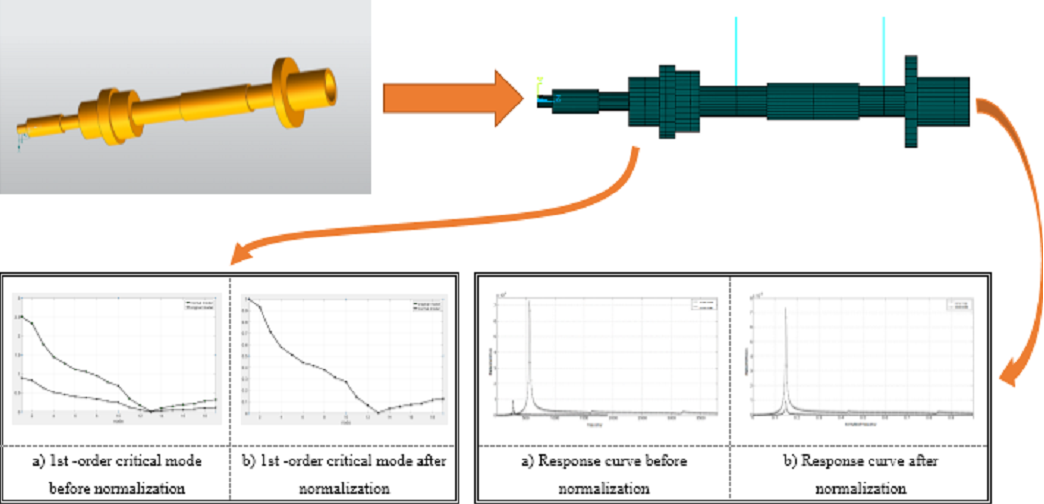## 1. Introduction

In terms of rotor dynamics test and dynamic similarity, many scholars have carried out a lot of useful explorations and achieved many results. Guoqiang H aimed at the simulation of the torsional vibration frequency of the rotor system, and used the physical structure similarity principle to design an analog rotor system similar to the physical structure of the 600 MW steam turbine shafting, The simulated rotor can better simulate the dynamic characteristics of the original steam turbine shaft . Paul designed a rotor tester for rotor dynamics research based on similarity theory . Guozhi Chen established a simulated rotor to simulate the dynamic characteristics of a gas generator rotor . Wu had analyzed the dynamic similarity of the scale model rotor and the actual rotor system based on the similarity theory. Which showed that the frequency of the scale model is proportional to the actual rotor, and the scale model can be used to predict vibration characteristics to the actual rotor . Zhongyong Gao applied the similarity theory to the design of the rotor tester. A simple rotor test model design method was proposed after the similarity of the model rotor and the actual rotor is discussed by the Ranlin’s formula and the matrix iteration formula . Luo had put forward the similar condition of model rotor and actual rotor after researched equation analysis method and dimensional analysis method .

The similarity criteria of the normal model and the original model for the rotor system is obtained by the dimensional analysis method in this paper. The critical speeds of the normal model and the original model were calculated by the finite element software ANSYS, and the similarity is verified.

## 2. Dimensional method

According to the structural characteristics of the rotor system and its differential equation of motion, the relevant physical quantities of the rotor model include: excitation force $F$, excitation frequency ${\omega }_{0}$, geometry size $l$, time $t$, elastic modulus $E$, stress $\sigma$, strain $s$, Poisson ratio $\mu$, damping ratio $\xi$, material density $\rho$, displacement response $U$, critical speeds $f$, bearing stiffness coefficient $k$ and bearing damping coefficient $c$.

For the normal vibration operation of the rotor, the relationship of the physical quantities of the rotor system in the linear elastic range can be expressed as:

1
$f\left(F,l,t,E,s,e,x,m,r,{w}_{0},f,U,k,c\right)=0.$

According to the- principle, $\pi$ criteria can be derived as follows:

2

In the similar model design of the rotor system, all physical quantities of the normal model and the original model must be proportional. The normal model and the original model are respectively represented by subscripts $m$ and $p$. It is assumed that geometrical dimensions ${l}_{m}$ and ${l}_{p}$ satisfy the similarity ratio ${C}_{l}$, the times ${t}_{m}$ and ${t}_{p}$ satisfy the similarity ratio ${C}_{t}$, the densities ${\rho }_{m}$ and ${\rho }_{p}$ satisfy the similarity ratio ${C}_{\rho }$, the elasticity modulus ${E}_{m}$ and ${E}_{p}$ satisfy the similarity ratio ${C}_{E}$, the excitation frequencies ${\omega }_{{0}_{m}}$ and ${\omega }_{{0}_{p}}$ satisfy the similarity ratio ${C}_{{\omega }_{0}}$, the excitation forces ${F}_{m}$ and ${F}_{p}$ satisfy the similarity ratio ${C}_{F}$, the displacement responses ${U}_{m}$ and ${U}_{p}$ satisfy the similarity ratio ${C}_{U}$, the velocity responses ${V}_{m}$ and ${V}_{p}$ satisfy the similarity ratio ${C}_{v}$, the acceleration responses ${a}_{m}$ and ${a}_{p}$ satisfy the similarity ratio ${C}_{a}$, and the support stiffness coefficients ${k}_{m}$ and ${k}_{p}$ satisfy the similarity ratio ${C}_{k}$, the bearing damping coefficients ${c}_{m}$ and ${c}_{p}$ satisfy the similarity ratio ${C}_{c}$, the scale formula can be obtained.

According to the scaling formula, the dynamic similarity of the normal model and the original model can be written as :

3

If the $r$-order mode of normal model and original model are ${\left\{{\psi }_{r}\right\}}_{m}$ and ${\left\{{\psi }_{r}\right\}}_{p}$ respectively, then ${\left\{{\psi }_{ir}\right\}}_{m}/{\left\{{\psi }_{ir}\right\}}_{p}={C}_{l}$. It can be seen that when the model size is fixed, there is a direct proportion of ${\left\{{\psi }_{r}\right\}}_{m}$ and ${\left\{{\psi }_{r}\right\}}_{p}$, and the modal shape is the complex amplitude ratio of each measurement point, substantially. If the modal shapes of two models are standardized on the elements in the same position, then:

4
${\left\{{\psi }_{r}\right\}}_{m}={\left\{{\psi }_{r}\right\}}_{p}.$

The modal shapes are exactly the same for the normal model and the original model in the same order.

In rotor model design, the normal model and original model are generally made of the same material, then ${C}_{E}={C}_{\rho }=$1. If the geometric scale is ${C}_{l}=\lambda$, then the physical quantities of normal model and the original model are shown in Table 1.

Table 1The scale of each physical quantity of normal model and original model of the rotor system

 Parameter Similarity ratio Proportion Feature length ${C}_{l}$ $\lambda$ Density ${C}_{\rho }$ 1 Elastic modulus ${C}_{E}$ 1 External force ${C}_{F}$ ${\lambda }^{2}$ Time ${C}_{T}$ $\lambda$ Excitation frequency ${C}_{{\omega }_{0}}$ 1/$\lambda$ Stiffness coefficient ${C}_{k}$ $\lambda$ Damping coefficient ${C}_{c}$ ${\lambda }^{2}$

## 3. Establishment of normal model of rotor system

As can be seen from Fig. 1. the original model consists of a rotating shaft and a disk, and two bearings with elasticity. Basic data of the original model is shown in Table 2. The finite element model of the rotor system is established according to the geometric model of the rotor system, as shown in Fig. 2. The rotating shaft is simulated by BEAM188, the disk is simulated by MASS21, and the bearing is simulated by COMBI214.

Fig. 1Structure of rotor system of original model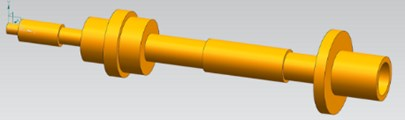Fig. 2Finite element model of the rotor system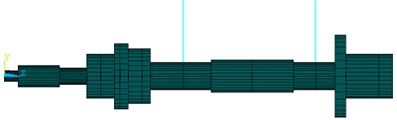Table 2Basic data of the original model

 Parameter Data Quality of the disk / kg 1.401 Moment of inertia about diameter / (kg·m3) 0.002 Polar moment of inertia / (kg·m3) 0.00136 Bearing stiffness coefficients / (N/m) 4.348×107 Elasticity modulus / GPa 207.8 Poisson’s ratio 0.3 Density / (kg/m3) 7806

In order to analyze the dynamic similarity between the normal model and original model, according to the similarity criteria, the normal model is established. All the relevant parameters shown in Table 3 are strictly in accordance with the similarity relation for the similarity transformation.

Table 3Basic data of the normal model of the rotor system

 Basic parameters Original model Normal model Geometric scale / $\lambda$ 1 0.5 Material density / (kg/m3) 7806 7850 Modulus of elasticity / (GPa) 207.8 207.8 Stiffness coefficient /(N/m) 4.378×107 2.189×107

## 4. Similarity analysis of dynamic characteristics of normal model and original model

The finite element models of the normal model and the original model are established respectively, and the modal analysis is carried out by QR method. The critical speeds of the normal model and the original model can be obtained with simulation analysis that has been shown in Table 4.

Table 4 and 5 show that the critical speeds of normal model are twice as much as that of the original model and the strain energy distribution is exactly the same.

Table 4The first three order critical speeds of normal model and original model

 Frequency order Original model / rpm 1/2 model / rpm Error / % 1st – order 17167 34335 0.0029 2nd – order 48988 97977 0.0010 3rd – order 95653 191305 0.0005

The modal shapes of the normal model of the rotor system can be obtained by using ANSYS. At the same time, the modal displacements of each order can be extracted and normalized. The modal displacement diagram of each node is shown in Fig. 3.

Table 5Strain energy distribution of the first 3rd order for the normal model and the original model

 Frequency order Original model Normal model Rotor Bearing I Bearing II Rotor Bearing I Bearing II 1st – order 47.4 44.8 7.8 47.4 44.8 7.8 2nd – order 26.1 0.40 73.5 26.1 0.40 73.5 3rd – order 64.7 31.1 4.2 64.7 31.1 4.2

Fig. 3Comparison and the first three critical modes of the original model and the normal model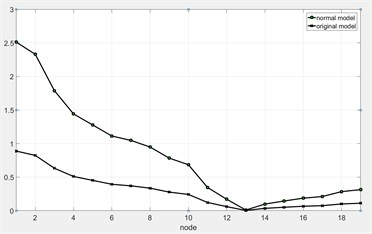a) 1st -order critical mode before normalization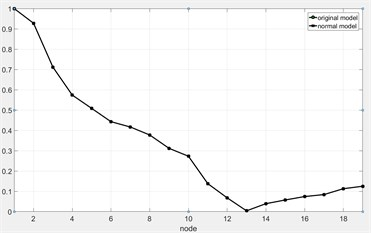b) 1st -order critical mode after normalization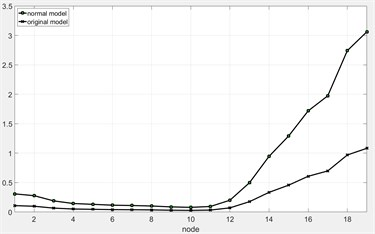c) 2nd -order critical mode before normalization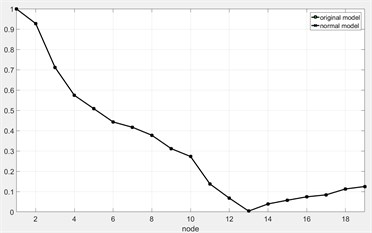d) 2nd -order critical mode after normalization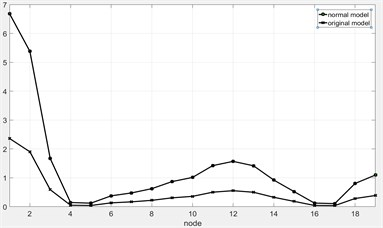e) 3rd -order critical mode before normalization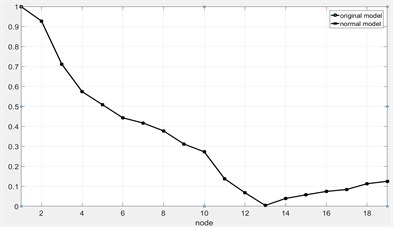f) 3rd -order critical mode after normalization

With the similarity analysis of the normal model and the original model for the rotor system, it can be found that dynamic characteristics of the normal model for the rotor system is similar to the dynamic characteristics of the original model. Therefore, the modal shapes and the critical speeds of the original model for the rotor system can be accurately predicted by the normal model.

In order to compare and analyze the similarity of the dynamic response for the normal model and the original model, the harmonic response analysis is carried out. With the harmonic response analysis, the steady-state response of the complex structure under the harmonic load can be determined. The damping effect need to be considered when studying the forced vibration response characteristics of the rotor. If the original model damping is 5 N/(m/s), and then the normal model damping is 25 N/(m/s) according to the similarity ratio.

The modal superposition method is used to analysis rotor harmonic response. The exciting forces applied to the normal model and the original model are 2.5 N and 10 N, respectively. With harmonic response analysis, the steady state response of each rotor system at the disk is obtained. The frequency response curves are shown in Fig. 4 and Fig. 5.

Fig. 4The frequency response curve of the normal model and the original model at the disk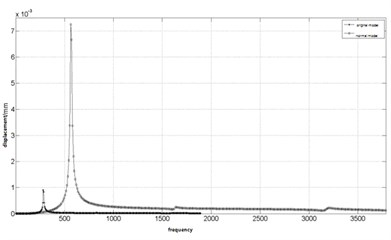a) Response curve before normalization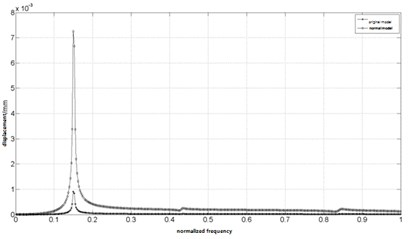b) Response curve after normalization

Fig. 5The frequency response curve of the normal model and the original model at the bearing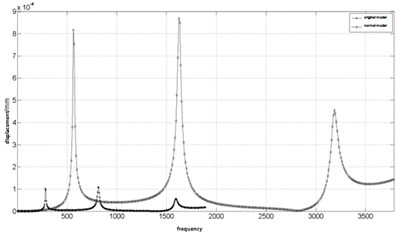a) Response curve before normalization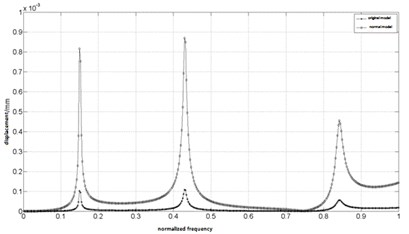b) Response curve after normalization

Fig. 4 and Fig. 5 show that the displacement-frequency curve of the normal model is basically similar to the curve of original model. It can be seen that the frequency dynamic response of two models are similar, so the normal model can accurately predict the frequency response characteristics of the original model subjected to external loads.

## 5. Conclusions

The normal similarity theory of the rotor system dynamic model was discussed in this paper. Based on the dimensional analysis method, the similarity criteria of the normal and original models for the rotor system were derived and the dynamic similarity relationship of two models were established. the dynamic characteristics and dynamic response similarity were analyzed by numerical simulation. the main conclusions show that:

1) The critical speeds of the normal and original models for the rotor system satisfy the determined relationship. in other words, if the geometrical size of the model is scaled down to 1/$n$ times of the original model, the critical speeds of the normal model will increase to n times of the original model. In addition, the vibration modes and the dynamic characteristics of the two models are completely identical.

2) With the application of similarity criteria, the amplitudes of response curves of the normal model is consistent with the amplitudes of the original model. in addition, dynamic response of two models are similar. Therefore, the normal model can accurately predict the forced vibration response characteristics of the original model under external loads.

Therefore, the normal model can accurately predict the dynamic vibration characteristics of the original model rotor system such as modal shapes, critical speeds and harmonic response.